# Acids and BasesPage 3

#### PREVIEW

Slide 17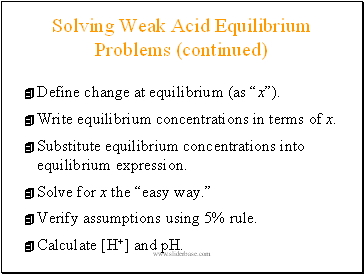Solving Weak Acid Equilibrium Problems (continued)

Define change at equilibrium (as “x”).

Write equilibrium concentrations in terms of x.

Substitute equilibrium concentrations into equilibrium expression.

Solve for x the “easy way.”

Verify assumptions using 5% rule.

Calculate [H+] and pH.

Slide 18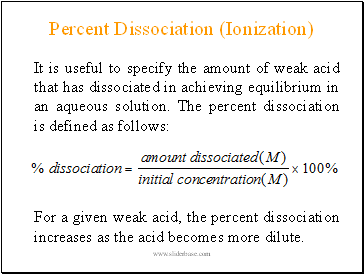## Percent Dissociation (Ionization)

It is useful to specify the amount of weak acid that has dissociated in achieving equilibrium in an aqueous solution. The percent dissociation is defined as follows:

For a given weak acid, the percent dissociation increases as the acid becomes more dilute.

Slide 19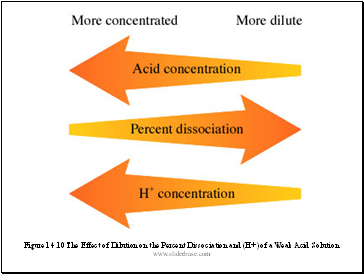Figure 14.10 The Effect of Dilution on the Percent Dissociation and (H+) of a Weak Acid Solution

Slide 20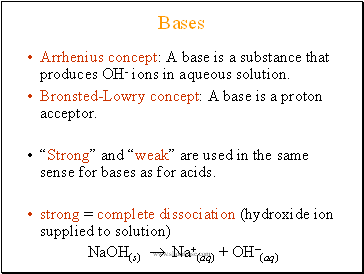## Bases

Arrhenius concept: A base is a substance that produces OH- ions in aqueous solution.

Bronsted-Lowry concept: A base is a proton acceptor.

“Strong” and “weak” are used in the same sense for bases as for acids.

strong = complete dissociation (hydroxide ion supplied to solution)

NaOH(s)  Na+(aq) + OH(aq)

Slide 21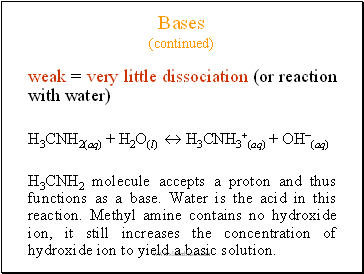Bases (continued)

weak = very little dissociation (or reaction with water)

H3CNH2(aq) + H2O(l)  H3CNH3+(aq) + OH(aq)

H3CNH2 molecule accepts a proton and thus functions as a base. Water is the acid in this reaction. Methyl amine contains no hydroxide ion, it still increases the concentration of hydroxide ion to yield a basic solution.

Slide 22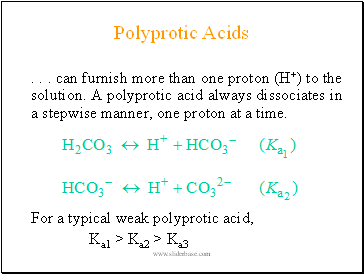## Polyprotic Acids

. . . can furnish more than one proton (H+) to the solution. A polyprotic acid always dissociates in a stepwise manner, one proton at a time.

For a typical weak polyprotic acid,

Ka1 > Ka2 > Ka3

Slide 23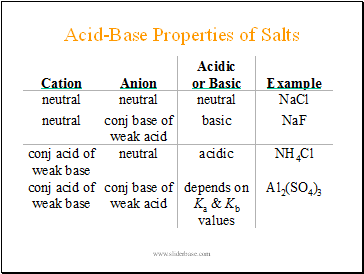## Acid-Base Properties of Salts

Slide 24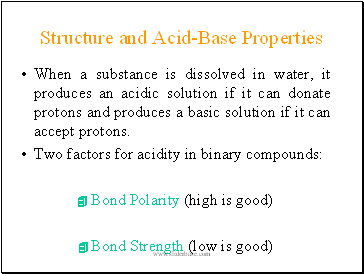## Structure and Acid-Base Properties

When a substance is dissolved in water, it produces an acidic solution if it can donate protons and produces a basic solution if it can accept protons.

Two factors for acidity in binary compounds:

Bond Polarity (high is good)

Bond Strength (low is good)

Slide 25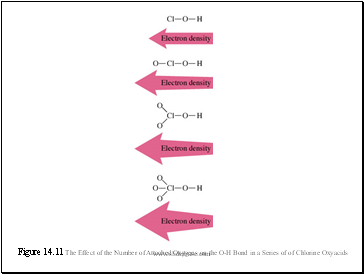Figure 14.11 The Effect of the Number of Attached Oxygens on the O-H Bond in a Series of of Chlorine Oxyacids

Go to page:
1  2  3  4